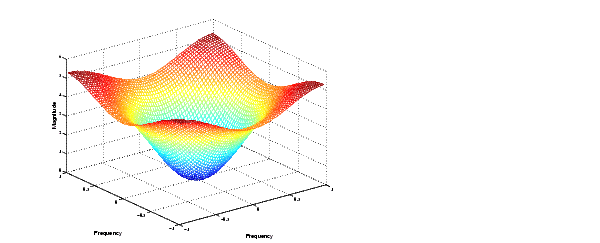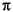Image Processing Toolbox User's GuideComputing the Frequency Response of a Filter

The `freqz2` function computes the frequency response for a two-dimensional filter. With no output arguments, `freqz2` creates a mesh plot of the frequency response. For example, consider this FIR filter,

• ```h =[0.1667    0.6667    0.1667
0.6667   -3.3333    0.6667
0.1667    0.6667    0.1667];
```

This command computes and displays the 64-by-64 point frequency response of `h`.

• ```freqz2(h)```

Frequency Response of a Two-Dimensional Filter

To obtain the frequency response matrix `H` and the frequency point vectors `f1` and `f2`, use output arguments

• ```[H,f1,f2] = freqz2(h);
```

`freqz2` normalizes the frequencies `f1` and `f2` so that the value 1.0 corresponds to half the sampling frequency, orradians.

For a simple `m`-by-`n` response, as shown above, `freqz2` uses the two-dimensional fast Fourier transform function `fft2`. You can also specify vectors of arbitrary frequency points, but in this case `freqz2` uses a slower algorithm.

See Fourier Transform for more information about the fast Fourier transform and its application to linear filtering and filter design.Creating the Desired Frequency Response Matrix Transforms